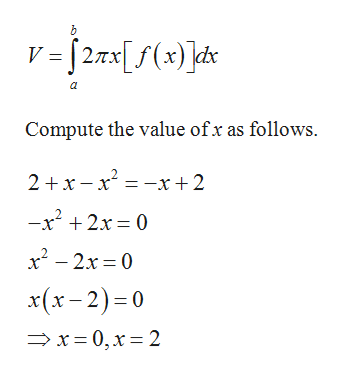Use the method of cylindrical shells to find the volume generated by rotating the region bounded by the curves y=2+x−x^2 and y+x=2 about the y-axis. Below is a graph of the bounded region.

Question

Use the method of cylindrical shells to find the volume generated by rotating the region bounded by the curves y=2+x−x^2 and y+x=2 about the y-axis. Below is a graph of the bounded region.

Step 1

The formula to calculate the volume using ...help_outlineImage Transcriptioncloseh V =[27x[f(x) ]ds a Compute the value ofx as follows. 2 x-x x+2 -x2 2x 0 x2-2x 0 x(x-2) 0 x-0,x 2 fullscreen

Want to see the full answer?

See Solution

Want to see this answer and more?

Our solutions are written by experts, many with advanced degrees, and available 24/7

See Solution
Tagged in

Other